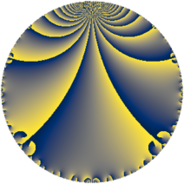# Properties

 Label 19.3.dLevel $19$ Weight $3$ Character orbit 19.d Rep. character $\chi_{19}(8,\cdot)$ Character field $\Q(\zeta_{6})$ Dimension $6$ Newform subspaces $1$ Sturm bound $5$ Trace bound $0$

# Related objects

## Defining parameters

 Level: $$N$$ $$=$$ $$19$$ Weight: $$k$$ $$=$$ $$3$$ Character orbit: $$[\chi]$$ $$=$$ 19.d (of order $$6$$ and degree $$2$$) Character conductor: $$\operatorname{cond}(\chi)$$ $$=$$ $$19$$ Character field: $$\Q(\zeta_{6})$$ Newform subspaces: $$1$$ Sturm bound: $$5$$ Trace bound: $$0$$

## Dimensions

The following table gives the dimensions of various subspaces of $$M_{3}(19, [\chi])$$.

Total New Old
Modular forms 10 10 0
Cusp forms 6 6 0
Eisenstein series 4 4 0

## Trace form

 $$6 q - 3 q^{2} - 9 q^{3} + 5 q^{4} - 2 q^{5} + q^{6} + 14 q^{9} + O(q^{10})$$ $$6 q - 3 q^{2} - 9 q^{3} + 5 q^{4} - 2 q^{5} + q^{6} + 14 q^{9} - 60 q^{10} + 26 q^{11} + 30 q^{13} + 54 q^{14} - 18 q^{15} + q^{16} - 42 q^{17} + 25 q^{19} + 108 q^{20} - 102 q^{21} - 39 q^{22} + 8 q^{23} - 83 q^{24} - 17 q^{25} - 148 q^{26} + 32 q^{28} - 12 q^{29} + 304 q^{30} + 51 q^{32} + 123 q^{33} - 6 q^{34} - 38 q^{35} - 54 q^{36} - 14 q^{38} - 44 q^{39} - 96 q^{40} + 63 q^{41} - 92 q^{42} - 34 q^{43} - 69 q^{44} - 28 q^{45} + 58 q^{47} - 147 q^{48} + 18 q^{49} + 132 q^{51} + 162 q^{52} - 12 q^{53} + 29 q^{54} - 28 q^{55} - 16 q^{57} + 172 q^{58} - 147 q^{59} - 222 q^{60} + 58 q^{61} - 116 q^{62} + 86 q^{63} + 166 q^{64} + 11 q^{66} + 201 q^{67} - 84 q^{68} - 198 q^{70} - 102 q^{71} + 210 q^{72} + 7 q^{73} + 174 q^{74} - 173 q^{76} - 376 q^{77} + 450 q^{78} + 134 q^{80} + 253 q^{81} - 145 q^{82} + 146 q^{83} - 90 q^{85} - 270 q^{86} - 568 q^{87} - 72 q^{89} - 438 q^{90} - 216 q^{91} + 72 q^{92} - 160 q^{93} + 558 q^{95} + 126 q^{96} + 21 q^{97} + 411 q^{98} - 56 q^{99} + O(q^{100})$$

## Decomposition of $$S_{3}^{\mathrm{new}}(19, [\chi])$$ into newform subspaces

Label Dim $A$ Field CM Traces $q$-expansion
$a_{2}$ $a_{3}$ $a_{5}$ $a_{7}$
19.3.d.a $6$ $0.518$ 6.0.6967728.1 None $$-3$$ $$-9$$ $$-2$$ $$0$$ $$q+(-1-\beta _{5})q^{2}+(\beta _{1}+2\beta _{2}+\beta _{3}+\beta _{5})q^{3}+\cdots$$